# Simplifying Formulas by Reducing the Complexity of IF Functions in Microsoft Excel 2010

In this article, we will learn simplifying formulas by reducing the complexity of IF functions in Microsoft Excel 2010.

While working on NESTED IF formula; the successful execution of the command depends on whether the condition is met or not. It is possible to nest multiple IF functions in a single cell.

IF function checks if the condition you specify is TRUE or FALSE. If the condition returns TRUE then it returns preset value, and if the condition returns FALSE then it returns another preset value.

Syntax = IF(logical_test,value_if_true,value_if_false)

logical_test: Logical test will test the condition or criteria. If the condition meets then it returns the preset value, and if the condition does not meet then, it returns another preset value.

value_if_true: The value that you want to be returned if this argument returns TRUE.

value_if_false: The value that you want to be returned if this argument returns FALSE

Using nested IF function will take too much time. We can use alternative function i.e. CHOOSE function to return the same output that IF function will return.

Choose: Returns the character specified by the code number from the character set for your computer. CHOOSE function will return a value from a list of values based on a given index number. Choose function uses index_num to return a value from a list.

Syntax =CHOOSE(index_num,value1,value2,...)

index_num: It specifies which value argument is selected. Index_num must be a number between 1 and 254 or a formula that contains the numbers between 1 and 254. If index_num is less than 1 then, choose will return #VALUE! error.

value1 & value 2 are 1 to 254 value arguments from which CHOOSE will evaluate & return the result.

Let us take an example:

• We have Students data table.
• In column A, we have Students name & in column B, we have their Ratings
• In column F & G, we have conditions- Score and Ratings.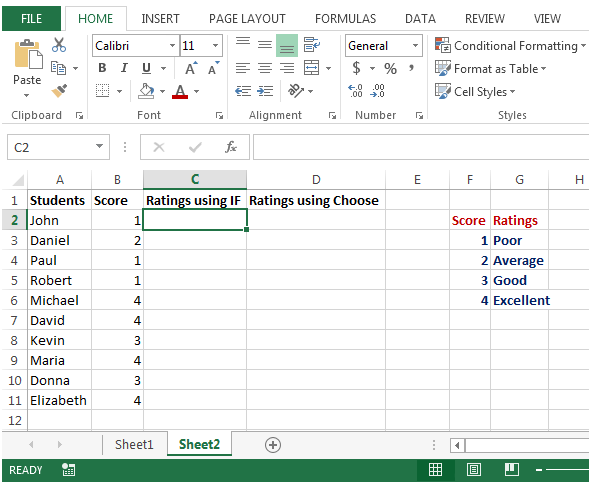• In column C, we want to enter nested IF formula that will return Ratings
• In cell C2, enter formula =IF(B2=1,\$G\$3,IF(B2=2,\$G\$4,IF(B2=3,\$G\$5,IF(B2=4,\$G\$6))))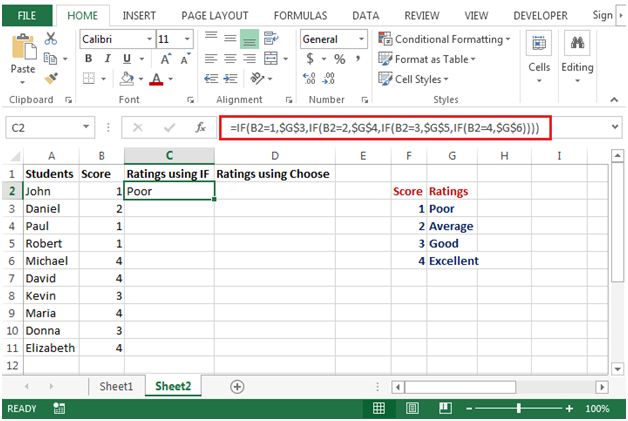• Copying down the formula below, we will get the output as below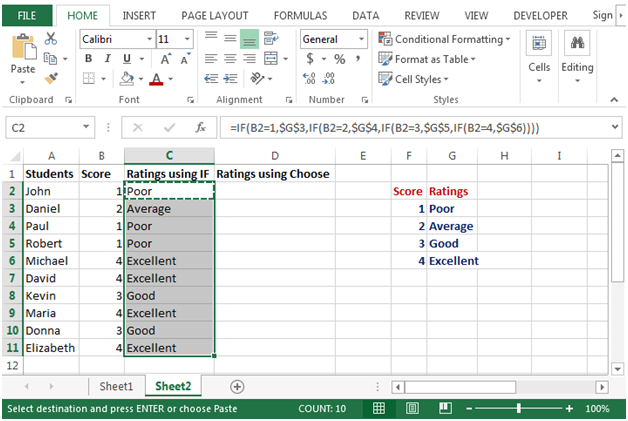• In cell D2, we will enter formula=CHOOSE(B2,\$G\$3,\$G\$4,\$G\$5,\$G\$6)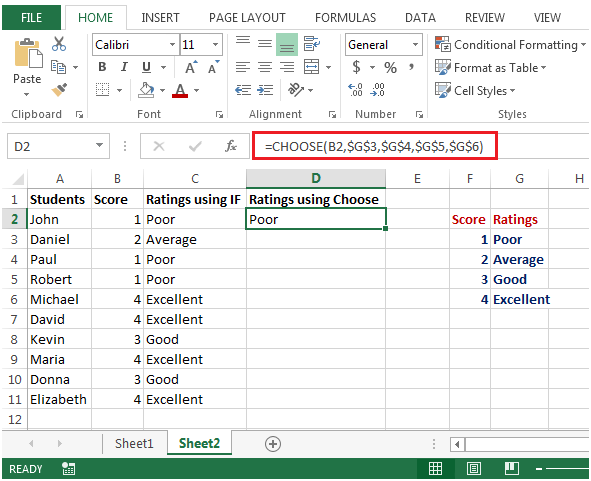• CHOOSE function will check value in cell B2 & return the value based on the values in column G.
• Copying down the formula below, we will get the output as below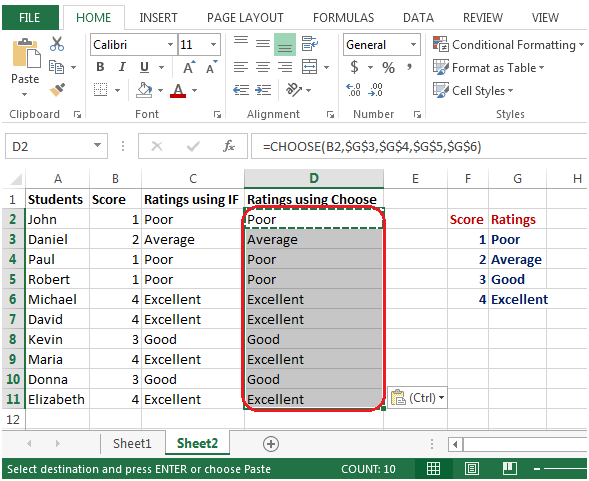• Both the formulas are providing the same output but using CHOOSE function that reduces complexity over IF function wherein for every new condition, we have to write a new IF condition within IF function.

Terms and Conditions of use

The applications/code on this site are distributed as is and without warranties or liability. In no event shall the owner of the copyrights, or the authors of the applications/code be liable for any loss of profit, any problems or any damage resulting from the use or evaluation of the applications/code.